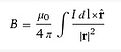## Biot-Savart law

The magnetic field at point P along the axis of a coil?Did we succeed scaring you off?Our intention is not to go into physics but only to elaborate a little on a common mistake made when explanations are given on figuring out the Gauss value at a specific distance from a PEMF  coil.

Contrary to popular belief that the so called inverse square law can be used to calculate how much intensity remains at a specific distance from a PEMF coil this is simply incorrect. This is only used as example to show that the farther you go away from the source of a magnetic field the less the magnetic intensity.

This is also easy to understand that when taking a regular magnet: the closer we come with the magnet to a metal surface the stronger the force attracting the magnet and the farther away the easier to remove the magnet from the metal surface.

The above mentioned inverse square law is applied for point source radiation for purposes like radar, X-rays, light and gravitation, and when it is used somewhere it's only as example for easy understanding what it means to be further away from a source, however for calculation of the magnetic field in a coil we need to make use of a different calculation method.

To calculate a magnetic field of a circular current loop at a point off-axis requires rather complex mathematics, so here is only the formula of the Biot-Savart law [which is the correct way to calculate the Gauss value at a specific distance from a coil] but we will not go into the actual calculations.Because electric current in a circular loop creates a magnetic field, by stacking multiple loops we create a so called solenoid without core, or what we call an air coil. To be able to calculate the magnetic field strength during the pulse into such a coil we need the Biot-Savart law where we can see in the formula that the radius of the coil r [which is half the diameter of the coil] is very important and critical for calculations, as will be explained below.

The smaller the coil the faster the magnetic intensity drops off when moving away from the coil, as shown in the

r-squared in the formula. Exactly this is the reason why the Curatron systems use large coils to minimize this effect and being able to completely penetrate the body even at a larger distance from the coils in the mattress.

This also explains that even when higher intensities are generated in a small coil, as soon as you get further away the faster the magnetic field drops off and the penetration depth becomes very small. Using an abundance of small coils in a mattress will not help penetration depth of the pulsing magnetic field as some manufacturers seem to think because they might not understand the Biot-Savart law for complicated magnetic fields.

Using coils with larger diameters however requires larger currents complicating design of PEMF devices. Having succeeded in also solving this problem clearly explains why Curatron systems are so extremely effective. It is even possible to feel the movements of the test magnet at the other side of the body proving that the Gauss value is still sufficiently high to completely penetrate through the body.